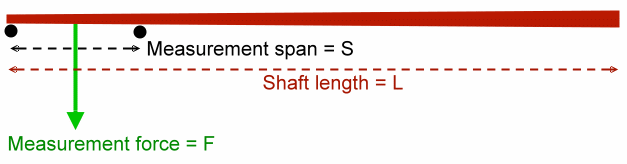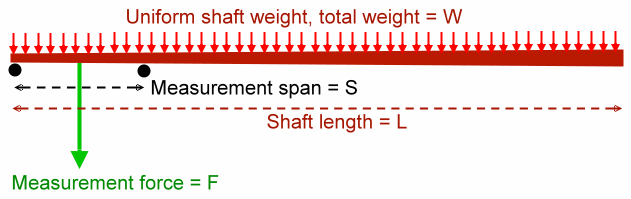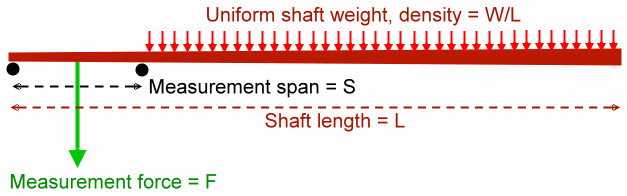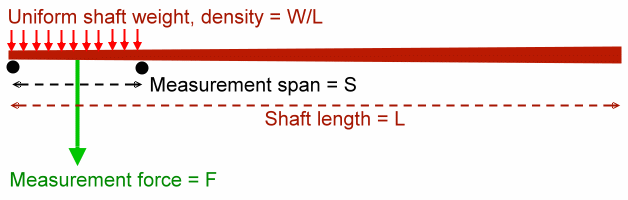# Errors in EI Measurement due to Shaft Weight

Dave Tutelman  --  August 28, 2011

Instruments that measure EI tend to use substantial forces to load the shaft, forces way more than the weight of the shaft. For that reason, the shaft weight is usually ignored, on the assumption that it is negligible compared to the loading force. Recently, Frans van Daalen brought to my attention a research paper from 1998 that found substantial measurement errors near the tip and butt due to the weight of the overhanging shaft section. This article finds an estimate for such errors.

Here are the major results, for those who don't want to wade through the math:
1. The error due to overhang for typical shafts may be as much as 8%. That is not a seriously large number, but is higher than most sources of error in EI measurement.
2. The error due to weight in the measured span is negligible, typically in the vicinity of 0.1%.
3. Such errors do not occur if you use differential deflection to do your measurements. That is, using both a pre-load and a full-load measurement, and subtracting one from another, will eliminate this source of error, and many others as well. Note that Russ Ryden's EI instrument, as well as mine and Don Johnson's, use differential deflection. If you use one of these designs, then shaft weight errors are of academic interest only. Why it happens is explained later in this article.

## AnalysisFigure 1. Idealized view of EI machine

Figure 1 shows the basic assumption for measuring EI, in this case, near the tip of the shaft. The shaft is supported at either side of a span of length S, and a measurement force F is applied at the middle of the span. The deflection due to the force is measured, and used to calculate EI. The calculation for deflection y is done using the well-known formula:

 y = FS3 48 EI

This is the idealized assumption for measuring EI. Note that the weight of the shaft is never considered. A more complete view of the measurement would consider shaft weight, and would look like Figure 2.Figure 2. More complete view includes shaft weight

Even this model is slightly idealized, assuming a uniform weight distribution over the length of the shaft. For most shafts, this is close to accurate. True, the wall thickness changes over the length of the shaft. But it tends to be thickest where the shaft has the largest diameter; the resulting weight distribution is much more uniform than you might imagine.

Considering shaft weight adds two sources of error:
• Overhang Error - The weight outside the span contributes a moment that will raise the point where deflection is mesured, reducing the measured deflection.
• Span Error - The weight inside the span contributes a load that will lower the point where deflection is measured, adding to the measured deflection.
The main work of this article is to estimate overhang and span errors. For our sample calculations, we choose values that tend to emphasize the error. A longer, heavier shaft gives more overhang error, and somewhat more span error. For the parameters for our computation, we will use:

 Parameter Value Comment L 48 inches Longer shaft gives more overhang error. W 80 grams Heavier shaft gives more overhang error. Also slightly more span error. S 10 inches Typical for EI machine. See article for pros and cons. F 20 pounds = 9100 grams This is a "middling" value for known EI machines. Higher values give less error, but risks deforming the shaft and introducing other forms of error.

Note that we are using a mixed system of units (inches and grams). It is worth worrying about, but in the end does not hurt us. Reasoning:
• We are consistent with our units for length (inches) and force (grams). Note that grams are force (not mass) in all our computations.
• Our final result is percentage error, which is dimensionless. It gets that way because every length is cancelled out (numerator and denominator), as is every force. So any conversion factor we needed to "fix" the units would also be canceled out. So the percentage error in this case is not affected by the mixed units.

### Overhang ErrorFigure 3. Overhang error

Figure 3 shows the factors involved in computing overhang error. We will compute the deflection yoverhang due to the overhanging weight. In this section, we will only consider the overhanging weight, nothing else. Then we will compare that deflection to our ideal deflection yideal. The result is the overhang error percentage.

The overhang weight contributes a moment -- and only a moment -- to the deflection in the middle of the span. Because we do not include any load in the span here, the bending moment is constant across the whole span.

The trick to computing this moment is to recognize that:
• The force acts through the middle of the overhang, so the moment arm is ½(L-S).
• The amount of the force is the weight of the overhang, the proportion of the shaft weight not over the span: W(L-S)/L.
So the moment M is

 M =  ½ (L-S) W (L-S) L = W(L-S)2 2 L

The shaft across the span is deflected by a constant moment, implying no shear at all. That is not a configuration in the usual handbooks, so we will have to derive it. We derive the deflection following the approach in Timoshenko's classic text. (S. Timoshenko, "Strength of Materials", Van Nostrand 1940, Chapter V) We start from the basic principle:

 EI d2y dx2 = -M

Since M is constant over the span, this should be simpler than Timoshenko's example. We start by multiplying both sides by dx and integrating:

 EI dy dx = -Mx + C

where C is the constant of integration. We evaluate C by knowing that the maximum deflection, and therefore zero slope, is at the middle of the span. So we set the slope dy/dx to zero at x=S/2.

0 = -MS/2 + C

C = MS/2

Thus:

 EI dy dx = -Mx + MS/2  =  M (S/2 - x)

So now we know the slope of the shaft between the spans. To get the deflection itself, we again multiply by dx and integrate.

 EI y  = M ( Sx 2 - x2 2 ) + C1

Once again, we need to evaluate the constant of integration. We are looking for maximum deflection at x=S/2, and the deflection is zero at x=0 and x=S. Let's set y=0 at x=0. (Note that we ought to get the same value for C1 if we had set y=0 at x=S, and that is exactly what happens.)

0 = 0 + C1
C1 = 0

Plugging this back into our last integration result:

 EI y  = M ( Sx 2 - x2 2 )

We want the deflection in the middle of the span, so set x=S/2 and solve for y.

 y  = M EI ( S2 4 - S2 8 )  = MS2 8 EI

Now all we have to do is plug in the formula we already computed for M.

 y  = S2 8 EI W(L-S)2 2 L = W S2(L-S)2 16 L EI

We're all set to compute the percentage error. That should be the overhang deflection computed here, divided by the ideal deflection computed earlier.

 yoverhang yideal = W S2(L-S)2 16 L EI * 48 EI FS3

 yoverhang yideal = 3W F (L-S)2 LS

Plugging in the values from our original table, we find that yoverhang / yideal is 0.079.

So the overhang error is 7.9%. Not an overwhelming amount, but still one of the largest sources of error in EI measurement.

(To assure myself that I did it right, I derived it another way and got the same answer. For those who are interested, the alternative derivation is in the Appendix.)

### Span ErrorFigure 4. Span error

Figure 4 shows the model for computing the span error. This turns out to be very easy, because there is a well-known formula for it.

 y  = 5 ρ S4 384 EI

The density ρ is already known to be W/L, so:

 y  = 5 W S4 384 L EI

As before, we will compute the percentage error. That should be the span deflection computed here, divided by the ideal deflection computed earlier.

 yspan yideal = 5 W S4 384 L EI * 48 EI FS3

 yspan yideal = 5 W 8 F S L

Plugging in the values from our original table, we find that yspan / yideal is 0.00114.

So the overhang error is about a tenth of a percent. Negligible!

## Why Differential Deflection Avoids Overhang Error

The statement is made above that differential deflection avoids overhang error completely (and span error, but that's negligible anyway). How does it do that?

Shaft bend is additive. If you separate out the bends due to each source and compute them separately, you can get the total bend by just adding them together. Engineers call this the Principle of Superposition, and use it all the time to compute elastic deformation. (In fact, we implicitly used it in the calculations above; now we will use it explicitly.) Superposition says that we can separately compute yideal, yoverhang, and yspan, and just add them together (signed addition, of course) to get the total deflection Y.

When we use differential deflection, we compare the actual measured deflection under two different conditions: with a pre-load and with a full load. Call these deflections Ypre and Yfull. So, in differential deflection, we actually measure twice.

Ypre  =  yideal(preload) + yoverhang + yspan

Yfull  =  yideal(full load) + yoverhang + yspan

Then we subtract Ypre from Yfull to get our working deflection. For our working load, we subtract the pre-load from the full load. Notice what happens:
• The deflection yoverhang is the same for both pre-load and full load, because it depends only on the weight of the shaft, not how much you load the shaft.
• Similarly for the deflection yspan, and for the same reason.
• So the subtraction causes yoverhang to cancel out, and likewise yspan, leaving only yideal(full load - preload).
So differential deflection measurements are immune to overhang and span error.

## Appendix

### Alternate derivation of overhang error

Timoshenko's treatment of bending starts with the observation that the bend at any point along the shaft is a curvature of some radius. The radius of curvature at that point on the shaft is given by EI/M (where M is, of course, the bending moment at that point on the shaft). But we are in the very fortunate position that overhang error results from a constant bending moment across the span S. Therefore, the bend is simply a circular arc of radius r. So let's deal with it as a geometry-trigonometry problem, rather than the calculus solution above.The figure to the right shows the geometry problem we are solving:
• The red arc is the shaft between the span supports (the black circles).
• The dashed line is a straight line across span S.
• There are three radii shown, from the center of curvature to the arc. Each is of the same length r because the bending moment is constant.
• The middle radius is divided into two segments, x and y, by the span line (dashed).
• Similarly, the span line is bisected into two halves, each of length S/2.
• Since the curvature is greatly exaggerated in this diagram (you'd never bend a shaft anywhere near this much to measure its EI), the angles a are in fact much smaller than shown. (This is important because we will be using small-angle approximations in the derivation.)
The deflection we want to find is y in the diagram. So let's start with:

y = r - x

We know that r is related pretty simply to the bending moment, but finding x is a geometry problem. We solve it by observing the trigonometry relations:

 sin a = S/2 r = S 2r

 cos a = x r

Now we are going to use the fact that a is a very small angle, so we can approximate sine and cosine as:

sin x ≅ x    and    cos x ≅ 1 - x2/2

Plugging these back into our equations for sine and cosine above, we get:

 a = S 2r

 1 - a2 2 =  1 - S2 2 * 4 r2 = x r

We can solve the last of these for x, which is what we are looking for.

 1 - S2 2 * 4 r2 = x r

 x  =  r - S2 8 r

Now we just apply this to our original proposition:  y = r - x

 y  =  r - x  =  r - r - S2 8r

 y  = S2 8r

Notice, it has just been a geometry problem so far, no physics. Now we use Timoshenko's bending curvature radius (r = EI/M), and plug it into the last equation:

 y  = M S2 8 EI

We already figured out the M due to overhanging weight.

 M = W(L-S)2 2 L

Plugging this into our equation for y:

 y  = W S2 (L-S)2 16 L EI

This is exactly the same as the equation for y that we got in the section on overhang error, so that answer is now more trusted.